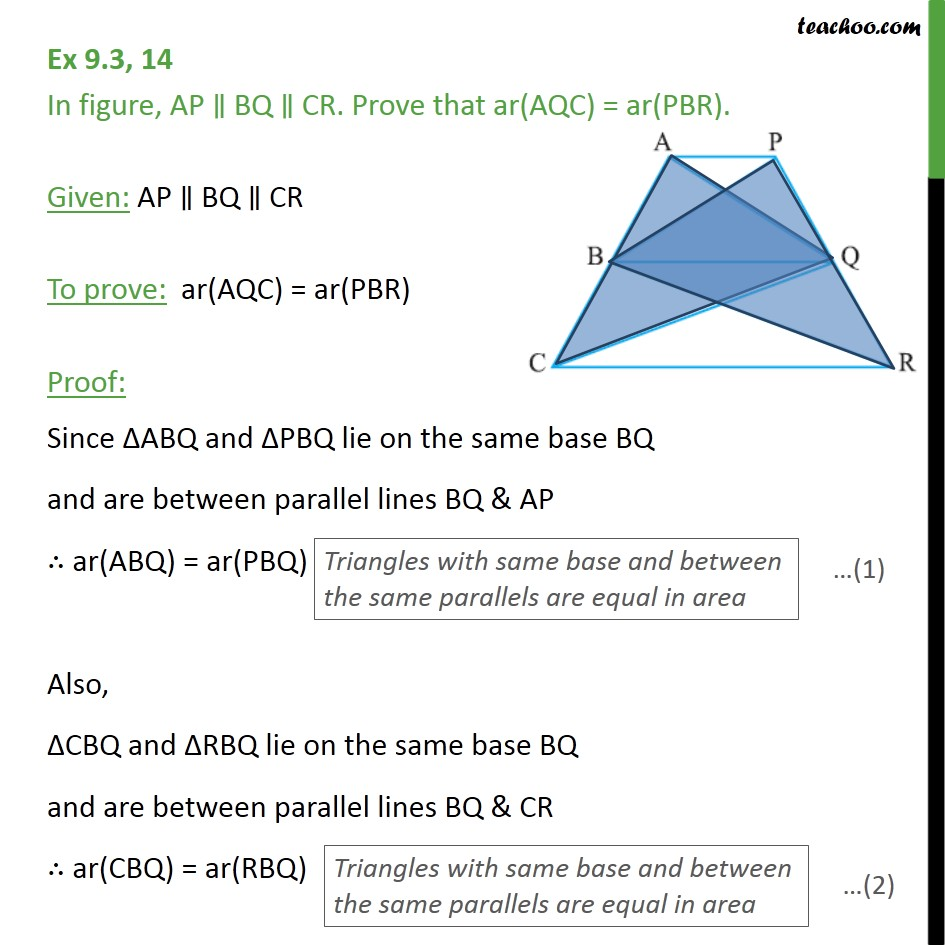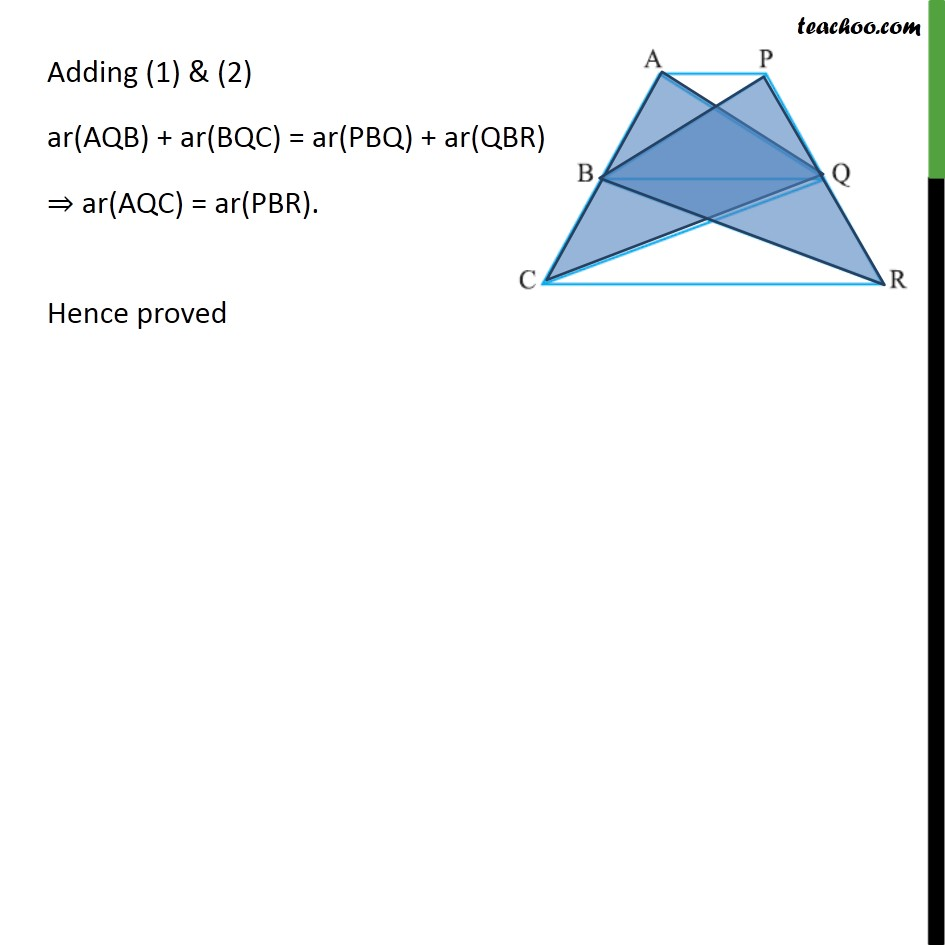Area of Triangles

Areas of Parallelograms and Triangles
Serial order wiseLearn in your speed, with individual attention - Teachoo Maths 1-on-1 Class

### Transcript

Question 14 In figure, AP BQ CR. Prove that ar(AQC) = ar(PBR). Given: AP BQ CR To prove: ar(AQC) = ar(PBR) Proof: Since ABQ and PBQ lie on the same base BQ and are between parallel lines BQ & AP ar(ABQ) = ar(PBQ) Also, CBQ and RBQ lie on the same base BQ and are between parallel lines BQ & CR ar(CBQ) = ar(RBQ) Adding (1) & (2) ar(AQB) + ar(BQC) = ar(PBQ) + ar(QBR) ar(AQC) = ar(PBR). Hence proved